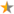# Template:Quest stage table

StageStatusDescription
Template documentation (for the template shown above, sometimes hidden or invisible)

## Contents

Template for quest stages in Gamebryo-engine games (Fallout 3, Fallout: New Vegas and their add-ons).

## Usage

```{{Quest stage table
|stage1   =
|status1  =
|desc1    =
|stage2   =
|status2  =
|desc2    =
...
|stage50  =
|status50 =
|desc50   =
}}
```

### Parameters

Sometimes required or at least one requiredstage1 up to stage50

Quest stage ID in G.E.C.K. ("Quest" edit window, "Quest Stages" tab, "Index" column).

Sometimes required or at least one requiredstatus1 up to status50

Quest status change (if any). Leave empty if no status change.

Available options:

• fail: This stage marks the quest as failed.
• finish: This stage marks the quest as finished.
Sometimes required or at least one requireddesc1 up to desc50

Description of quest stage (i.e. explanation when this stage is set).

## Example

```{{Quest stage table
|stage1   =5
|status1  =
|desc1    =Player has been tasked to make a template.
|stage2   =10
|status2  =fail
|desc2    =The template is not working.
|stage3   =15
|status3  =finish
|desc3    =Player has finished the template.
}}
```

results in:

StageStatusDescription
5 Player has been tasked to make a template.
10Quest failedThe template is not working.
15Quest finishedPlayer has finished the template.

## Copy/paste

More than 30 will rarely be needed; expand if necessary.

```{{Quest stage table
|stage1   =
|status1  =
|desc1    =
|stage2   =
|status2  =
|desc2    =
|stage3   =
|status3  =
|desc3    =
|stage4   =
|status4  =
|desc4    =
|stage5   =
|status5  =
|desc5    =
|stage6   =
|status6  =
|desc6    =
|stage7   =
|status7  =
|desc7    =
|stage8   =
|status8  =
|desc8    =
|stage9   =
|status9  =
|desc9    =
|stage10  =
|status10 =
|desc10   =
|stage11  =
|status11 =
|desc11   =
|stage12  =
|status12 =
|desc12   =
|stage13  =
|status13 =
|desc13   =
|stage14  =
|status14 =
|desc14   =
|stage15  =
|status15 =
|desc15   =
|stage16  =
|status16 =
|desc16   =
|stage17  =
|status17 =
|desc17   =
|stage18  =
|status18 =
|desc18   =
|stage19  =
|status19 =
|desc19   =
|stage20  =
|status20 =
|desc20   =
|stage21  =
|status21 =
|desc21   =
|stage22  =
|status22 =
|desc22   =
|stage23  =
|status23 =
|desc23   =
|stage24  =
|status24 =
|desc24   =
|stage25  =
|status25 =
|desc25   =
|stage26  =
|status26 =
|desc26   =
|stage27  =
|status27 =
|desc27   =
|stage28  =
|status28 =
|desc28   =
|stage29  =
|status29 =
|desc29   =
|stage30  =
|status30 =
|desc30   =
}}
```
Documentation transcluded from Template:Quest stage table/doc.